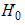# NMath User's Guide

42.6 Significance of the Overall Model (.NET, C#, CSharp, VB, Visual Basic, F#)

Class tests the overall model significance for linear regressions. Simply construct a LinearRegressionAnova from a LinearRegression object:

Code Example – C# linear regression

```var lrAnova = new LinearRegressionAnova( lr );
```

Code Example – VB linear regression

```Dim LRAnova As New LinearRegressionAnova(LR)
```

A variety of properties are provided for assessing the significance of the overall model:

RegressionSumOfSquares gets the regression sum of squares. This quantity indicates the amount of variability explained by the model. It is the sum of the squares of the difference between the values predicted by the model and the mean.

ResidualSumOfSquares gets the residual sum of squares. This is the sum of the squares of the differences between the predicted and actual observations.

ModelDegreesOfFreedom gets the number of degrees of freedom for the model, which is equal to the number of predictors in the model.

ErrorDegreesOfFreedom gets the number of degress of freedom for the model error, which is equal to the number of observations minus the number of model paramters.

RSquared gets the coefficient of determination.

MeanSquaredResidual gets the mean squared residual. This quantity is the equal to ResidualSumOfSquares / ErrorDegreesOfFreedom (equals the number of observations minus the number of model parameters).

MeanSquaredRegression gets the mean squared for the regression. This is equal to RegressionSumOfSquares / ModelDegreesOfFreedom (equals the number of predictors in the model).

FStatistic gets the overall F statistic for the model. This is equal to the ratio of MeanSquaredRegression / MeanSquaredResidual. This is the statistic for the hypothesis test where the null hypothesis,is that all the parameters are equal to 0 and the alternative hypothesis is that at least one paramter is nonzero.

FStatisticPValue gets the p-value for the F statistic.

For example:

Code Example – C# linear regression

```var lrAnova = new LinearRegressionAnova( lr );
```
```double sse = lrAnova.ResidualSumOfSquares;
```
```double r2 = lrAnova.RSquared;
```
```double fstat = lrAnova.FStatistic;
```
```double fstatPval = lrAnova.FStatisticPValue;
```

Code Example – VB linear regression

```Dim LRAnova As New LinearRegressionAnova(LR)
```
```Dim SSE As Double = LRAnova.ResidualSumOfSquares
```
```Dim R2 As Double = LRAnova.RSquared
```
```Dim FStat As Double = LRAnova.FStatistic
```
```Dim FStatPVal As Double = LRAnova.FStatisticPValue
```

Lastly, the FStatisticCriticalValue() function computes the critical value for the F statistic at a given significance level:

Code Example – C# linear regression

```double critVal = lrAnova.FStatisticCriticalValue(.05);
```

Code Example – VB linear regression

```Dim CritVal As Double = LRAnova.FStatisticCriticalValue(0.05)

```

Top

Top